A Source Book in Astronomy and Astrophysics, 1900-1975

Contents:
Author: Ralph Howard Fowler  | Date: 1926

Show Summary

## On Dense Stars

Ralph Howard Fowler

(Monthly Notices of the Royal Astronomical Society 87, 114–122 )

### 1. INTRODUCTION

THE ACCEPTED DENSITY of matter in stars such as the companion of Sirius is of the order of 105 gm./c.c. This large density has already given rise to most interesting theoretical considerations, largely due to Eddington. We recognise now that matter can exist in such a dense state if it has sufficient energy, so that the electrons are not bound in their ordinary atomic orbits of atomic dimensions, but are in the main free—with sufficient energy to escape from any nucleus they may be near. The density of such "energetic" matter is then only limited a priori by the "sizes" of electrons and atomic nuclei. The "volumes" of these are perhaps 10-14 of the volume of the corresponding atoms, so that densities up to 1014 times that of terrestrial material may not be impossible. Since the greatest stellar densities are of an altogether lower order of magnitude, the limitations imposed by the "sizes" of the nuclei and electrons can be ignored in discussions of stellar densities, and the structural particles of stellar matter can be treated as massive charged points.

Eddington has recently1 pointed out a difficulty in the theory of such matter. Assuming it to behave more or less like a perfect gas, modified by its electrostatic forces and the sizes of such atomic structures as remain undissolved, there is a perfectly definite relation between the energy and the temperature, which depends on the density only to a minor degree. This assumption even here is not so unreasonable as appears at first sight. But even without it we naturally expect a perfectly definite relation between energy and temperature, in which there is a close correlation between large energies and large temperatures, small energies and small temperatures. The emission of energy by the star will proceed in the usual way at a rate depending on the surface temperature, and the internal temperatures must provide the gradient necessary to drive the radiation out. So long as the star contains matter at a high temperature, radiation of energy must presumably go on. But then, according to Eddington, there may come a time when a very curious state of affairs is set up. The stellar material will have radiated so much energy that it has less energy than the same matter in normal atoms expanded at the absolute zero of temperature. If part of it were removed from the star, and the pressure taken off, what could it do?

The present note is devoted to a further consideration of this paradox. It is clear that the crucial point is the connection between the energy and the temperature. In a sense the temperature measures the "looseness" of the system, the number of possible configurations which it can assume, and therefore its radiation. These depend directly on the temperature, and only on the energy in so far as the energy determines the temperature. The excessive densities involved suggest that the most exact form of statistical mechanics must be used to discuss the relationship between the energy, temperature, and density of the material. This is a form suggested by the properties of atoms and the new quantum mechanics, which has been already applied to simple gases by Fermi and Dirac.2 It may be accepted now as certain that classical statistical mechanics is not applicable at extreme densities, even to ideal material composed of extensionless mass-points, and that the form used here is fairly certainly the correct substitute. Its essential feature is a principle of exclusion which prevents two mass-points ever occupying exactly the same cell of extension h3 of the six-dimensional phase-space of the mass-points. When this form of statistical mechanics is adopted, it at once appears that the suggested difficulty resolves itself, and there is really no difficulty at all. The apparent difficulty was due to the use of a wrong correlation between energy and temperature, suggested by classical statistical mechanics. When the correct relation is substituted, it is found that the limiting state of such dense stellar matter is one which the energy is still, as it must be, excessively great, but the temperature is zero! Since the temperature determines the radiation, radiation stops when the dense matter has still ample energy to expand and form normal matter if the pressure happened to be removed. As the dense matter radiates its energy away, the number of its possible configurations rapidly falls, and therewith the temperature. The absolutely final state is one in which there is only one possible configuration left. Temperature then ceases to have any meaning, for the star is strictly analogous to one gigantic molecule in its lowest quantum state. We may call the temperature then zero.

Whether or no some such explanation may not be equally possible using other forms of statistical mechanics (perhaps the classical) I am not prepared to say. The new form used here seems for entirely independent reasons so satisfactory that its applicability need not be questioned. On application it clears up Eddington’s question in a convincing manner, and I am content to leave the matter so.

### 2. THE EQUILIBRIUM STATE OF DENSE MATTER

It is obviously reasonable to consider dense stellar material as an assembly of free electrons and bare nuclei of net charge zero. It is, however, necessary to idealise the problem a little further and ignore the electrostatic charges, so that the whole energy of the assembly is the kinetic energy of translation of the various particles. We shall later see reason to believe that this idealisation does not vitiate the results, or at least not their qualitative form, for we are really mainly concerned with calculations of amounts of phase-space, and these are independent of potential energy.

Consider a volume V of stellar material consisting of N/q bare nuclei of mass mq and N free electrons of mass m, with total (kinetic) energy E. We will assume as a first approximation, as we have said, that the electrostatic forces may be ignored, and then that the statistics of assemblies of practically independent systems is applicable. Then3 the total number of complexions C representing any state of the assembly is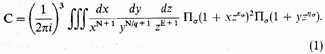In this equation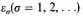are the possible energies of an independent electron in a volume V, and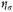those of an independent nucleus. The power 2 enters because the electron has a structure (axis of spin) which gives it two possible orientations in a magnetic field, that is, a statistical weight 2. The nucleus probably also has a weight greater than unity, but the nuclear terms turn out to be unimportant, so that we need not spend time over this refinement. Students of classical statistical mechanics will recall that the classical formula differs from this only in having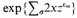in place of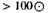All the mean values associated with the equilibrium state of the assembly can be derived from C, and the value of C can be obtained by "steepest descents." Writing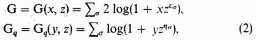the critical value of the integrand is determined by the unique root λ, μ, θ of the equations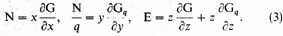With these special values of x, y, z we have, among other equations,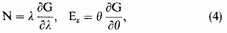where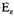is the average energy of the free electrons. Also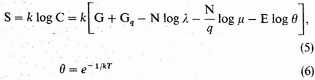The number of possible energy values for an electron of mass m in a volume V which lie between τ and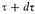is4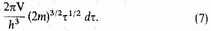Therefore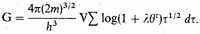The values of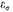are extremely closely spaced, so that for a fixed value of θ we can replace the sum by an integral with negligible error and find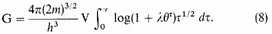Strictly we shall want to make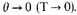The form (8) might then fail, but as we shall really not be interested in the differences between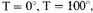or even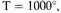this does not matter, and we shall assume the general validity of (8). The validity of some applications of (8) can be checked in the limit by direct calculation. N and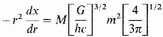are obtained from this by differentiation. Similar forms and equations hold for Gq, N/q, and Eq for the nuclei. The assembly is a thermodynamic system.

Our chief interest is now to trace the series of equilibrium states of such an assembly as its energy is slowly radiated away. A loss of energy by radiation means necessarily a loss of entropy, and therefore a fall of temperature. We have therefore to trace the behavior of the assembly as θ decreases—presumably as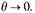But by (4) and (8) N depends on a function of the form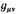If λ has an upper bound b, this is less than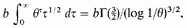and therefore tends to 0 as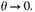This would make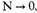which is impossible, as the number of particles is fixed. Since λ cannot have an upper bound, it is reasonable to assume that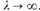We shall see shortly that this is a necessary consequence of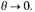Let us therefore write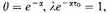defining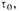and assume that λ and therefore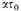are large. Then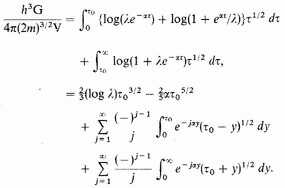In transforming the integrals we have used the fact that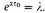Since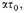and a fortioriare large, we have approximately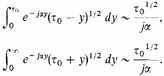Therefore finally (and approximately)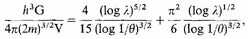an equation whose real value can be expressed in the form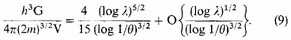This equation can be differentiated. Therefore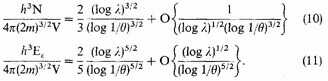It follows from (10) that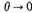implies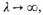and therefore from (10) and (11) combined that as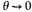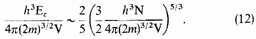The temperature can (and presumably would) fall to zero, still leaving, however, the free electrons with kinetic energy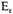given by (12). Similar relations hold for the nuclei. In the limit, however,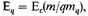and is therefore negligible compared with.

It is wise to check up the behaviour of the entropy meanwhile. The contribution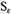by the electrons is given by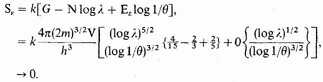The contribution by the nuclei behaves in a similar way. This is correct.

We may note that the relation (12) can be confirmed by a direct calculation of the tight-packed phase-space required to house the N electrons. If the number of cells is the number of electrons, we find from (7)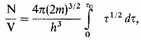while at the same time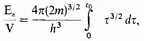which together give (12).

### 3. NUMERICAL CALCULATIONS

Let us assume that the density of the stellar matter is 10x gm./c.c., and that it is composed on the average of iron atoms, at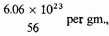or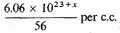Then for the free electrons,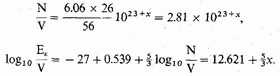For density 105 gm./c.c.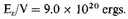Thus the average kinetic energy of an electron in this state is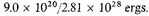This is the same as the average kinetic energy of an electron in an expanded gas at a temperature T, where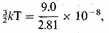or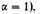It is not, however, possible to rest quite content with this result, since the electrostatic energy terms are far from negligible, and in fact of the same order of magnitude. We observe first that the equation (12) is based entirely on considerations of phase-space. There must be enough cells of extension h3 in six-dimensional phase-space to accommodate all the electrons. The phase-space depends only on velocities, or kinetic energy of the electrons, and is independent of the potential energy terms. The relation (12), therefore, should continue to give the necessary kinetic energy of the electrons, at least reasonably accurately, even when the effects of potential energy are allowed for. We have therefore merely to attempt to estimate the negative potential energy in order to compare the total energy of the condensed matter with its total energy in an expanded form.

The negative potential energy of a normal atom can be estimated roughly but easily if we recall that the average negative potential energy of an orbit in an inverse square field is twice the negative (total) energy of the orbit. The total negative energy of an iron atom is5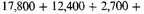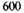volts for the K, L, M, and N electrons respectively, or 33,500 volts in all. The negative potential energy of one atom may therefore be taken to be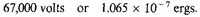The negative potential energy of the atoms equivalent to 1 c.c. of density 10x is therefore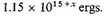For the standard case of density 105 the negative energy of the normal atoms is, therefore, say 1020 ergs.

The negative potential energy in the condensed form is far more difficult to estimate with any pretence to accuracy. If we simply say that at a density 10x everything will on the whole be about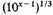closer than it could be packed before in normal atoms, we might argue that the negative potential energy would be increased about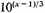times, and so become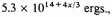or in the standard case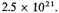This, however, must grossly over-estimate the increase. For half the negative energy comes from the two K electrons normally at a distance of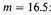from the nucleus. The average distance of uniformly distributed electrons at a density 105 is as much as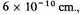and there is no reason for two electrons on the average to sink into about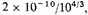that is 10-11 cm. They might be expected to get hardly any closer, and the L-electrons not to experience the full shrinkage. If the contribution of the two electrons corresponding to the K electrons is unaltered, the potential energy increase, and therefore roughly the potential energy, is halved, and the incomplete shrinkage of the L group would halve the energy again. A negative potential energy of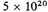is all that can be expected.

An alternative estimate may be made as follows. The average space assigned to each nucleus is a sphere of radius r0, where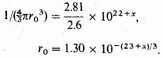Let us estimate the electrostatic energy by distributing 26 electrons uniformly between ο and r0 for each nucleus, assume that the field of a single point charge 26e acts on each and ignore their own repulsions. Such an average distribution is not far wrong, for it is known that there is comparatively little crowding of bound electrons owing to the quantum restrictions, or of free electrons owing to the energy conditions.6 Thus the negative energy per atom is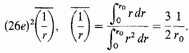The negative potential energy per c.c. of condensed matter is therefore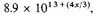or in the standard case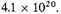All this is very uncertain; the best we can do is to conclude that the negative potential energy of the condensed form is about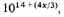or in the standard case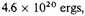compared with 1020 ergs when expanded in normal atoms. As a result the kinetic energy is perhaps twice the negative potential energy for a density 105, and the expanded form could become a perfect gas of normal atoms at about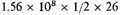say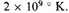Whether or no by our rough calculations we can show that the total energy of the condensed form is large and positive for a density of 105, we can certainly conclude that this must be true for still greater densities, for the kinetic energy per unit volume varies as 10(5x/3), and on almost any view the negative potential energy must vary ultimately like 10(4x/3). There seems no reason to doubt that this inequality, kinetic energy greater than negative potential energy, is true over wider conditions than we can show by these arguments. For in condensed forms at somewhat lower densities the tight packing is presumably achieved at the expense of shifting only the more lightly bound electrons, and our estimates of increase in the negative potential energy will be greatly in excess.

### 4. COGNATE SPECULATIONS

If we return to the condensed assembly of mass-points, electrostatic forces ignored, we may note that the pressure is given by the thermodynamic equation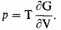This is strictly the pressure exerted by the electrons, and obeys the usual perfect gas equation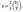the contribution by the nuclei is trivial by comparison. Thus in the condensed limit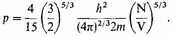If the volume is a sphere of radius R, then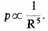This pressure represents an interaction of the assembly with the outside world which is in a sense due to the quantum restraints to which the assembly is subject.

Now an atom, especially an atom of an inert gas, is really condensed matter of just the same general type. The assembly, whether atom or stellar material, contains just so many cells of phase-space available for electrons and every one of them is full. It is tempting to speculate as to whether the apparent forces of repulsion, which prevent the too close approach of atoms, molecules, or ions with closed configurations of electrons, may not be due in just the same way purely to the quantum constraints. The number of cells of the phase-space must be unaltered in an encounter, and the apparent force of repulsion represents just the work which must be done to make up by an increase in the velocity (or kinetic energy) space for a loss in the extent of the "space"-space. These forces of repulsion between condensed atomic configurations of electrons seem empirically to vary like 1/R10, or there-abouts, for a large variety of atoms. It would be too much to expect the pressure of the stellar material to follow exactly the same law. But the general forms of the two types of system are sufficiently alike, and the resemblance of their laws of interaction close enough to lead one to believe that the origin of this important part of inter-atomic forces is to be sought in this direction, in the quasi-thermodynamic consequences of the existence of the quantum constraints embodied in Pauli’s principle.

1. E. C. Stoner, Philosophical Magazine 7, 63 (1929), 9, 944 (1930); W. Anderson, Zeitschrift für Physik 54, 433 (1929).

2. S. Chandrasekhar, Astrophysical Journal 74, 81 (1931), Monthly Notices of the Royal Astronomical Society 95, 207, 226, 676 (1935), and An Introduction to Stellar Structure (Chicago: University of Chicago Press, 1938).

1. Eddington, The Internal Constitution of the Stars (Cambridge Univ. Press, 1926), §117.

2. Fermi, Rend. Acc. Lincei, Ser. 6, 3, 145 (1926); Dirac, Proc. Roy. Soc. A 112, 661 (1926). See also Fowler, Proc. Roy. Soc. A 113, 432 (1926), for a full account with other references.

3. The following formulæ will be found in detail in Fowler.

4. Dirac, p. 671.

5. Hartree, Proc. Camb. Phil. Soc. 22, 464 (1924).

6. For the latter see Eddington, §183.

Contents:

### Related Resources

None available for this document.

Title: A Source Book in Astronomy and Astrophysics, 1900-1975

Select an option:

*Note: A download may not start for up to 60 seconds.

## Email Options

Title: A Source Book in Astronomy and Astrophysics, 1900-1975

Select an option: